# Units - math word problems

#### Number of problems found: 3975

• Magic bagEach time the prince crossed the bridge, the number of tolars in the magic bag doubled. But then the devil always conjured 300 tolars for him. When this happened for the third time, the prince had twice as much as he had in the beginning. How many tolars
• The basesThe bases of the isosceles trapezoid ABCD have lengths of 10 cm and 6 cm. Its arms form an angle α = 50˚ with a longer base. Calculate the circumference and content of the ABCD trapezoid.
• GlassHow many glass are needed to produce glass with base regular 5-gon if one base triangle in the base is 4.2 square cm and the height is 10 cm?
• Car and cyclist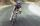Cyclist started from town at a speed of 18 km/h. After 1 hour 30 min started the car from town and caught up with the cyclist in 50 minutes. How fast was a car?
• Tractors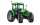Wheel and belt tractors each day plow 8 ha of fields. For plow 76 hectares, the wheel would have to work for 12 days and 8 days with the belt tractor together. How many hectares do each day of each tractor?
• Train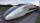A passenger train traveled for 2 hours 74 km. 3.1 hours after its departure started fast train and caught it on 186 km. How many km/h is different its average speeds?
• Hexagonal pyramidRegular hexagonal pyramid has dimensions: length edge of the base a = 1.8 dm and the height of the pyramid = 2.4 dm. Calculate the surface area and volume of a pyramid.
• Cube containers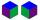Two containers shaped of cube with edges of 0.7 m and 0.9 m replace a single cube so that it has the same volume as the original two together. What is the length of the edges of the new cube?
• Inclined plane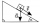The body stays on an inclined plane and exerts a compressive force of 70N on it. Find the angle between the inclined plane and the horizontal if a gravitational force of 100N acts on the body.
• Trapezium diagonalsIt is given trapezium ABCD with bases | AB | = 12 cm, |CD| = 8 cm. Point S is the intersection of the diagonals for which |AS| is 6 cm long. Calculate the length of the full diagonal AC.
• A concrete pedestalA concrete pedestal has a shape of a right circular cone having a height of 2.5 feet. The diameter of the upper and lower bases are 3 feet and 5 feet, respectively. Determine the lateral surface area, total surface area, and the volume of the pedestal.
• Imagine 2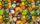Imagine that you are filling treat bags with jellybeans. You have 3 3/8 cups of jellybeans, and each treat bag will contain 1/4 cup of jellybeans. How many treat bags can you fill before you run out of jellybeans? Show your work (please).
• Tourist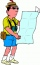A tourist walked an average speed of 3.5 km/h route in 6 hours. Calculate how many hours he would have passed at an average speed of 5.5 km/h.
• Gasoline canisters35 liters of gasoline is to be divided into 4 canisters so that in the third canister will have 5 liters less than in the first canister, the fourth canister 10 liters more than the third canister and the second canister half of that in the first canister
• TailorTailor has an estimated 11 meters of 80 cm wide fabric. He will shape wholly 7 rectangles measuring 80 x 150 cm and nothing fabric does not remain. How many fabric he have?
• Office rent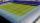For rent 1m2 of office space is paid €165 per year. How much is the annual rent for an office as big as a football field with dimensions 71.5 m and 102.5 m?
• Daily temperature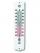The average of daily temperature measurements in one week every day at the same hour was -2.8 °C. We measured all temperatures on different days are different. The highest daily maximum temperature was 2.4 °C, the lowest -6 °C. Determine the options that
• Lampshade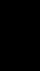The cone-shaped lampshade has a diameter of 30 cm and a height of 10 cm. How many cm2 of material will we need when we 10% is waste?
• Rope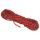Half of the rope used Mam for packaging, half of the rest got a son, half of the rest he took the father and two-fifths of what remained, and got a daughter. Remained 143 cm of rope. How long was the rope at the beginning?
• A squareA square with a length of diagonals 12cm give: a) Calculate the area of a square b) rhombus with the same area as the square, has one diagonal with a length of 16 cm. Calculate the length of the other diagonal.

Do you have an interesting mathematical word problem that you can't solve it? Submit a math problem, and we can try to solve it.

We will send a solution to your e-mail address. Solved examples are also published here. Please enter the e-mail correctly and check whether you don't have a full mailbox.

Please do not submit problems from current active competitions such as Mathematical Olympiad, correspondence seminars etc...